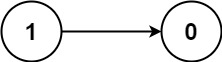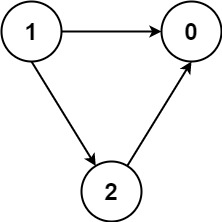# GeetCode Hub

There are a total of `numCourses` courses you have to take, labeled from `0` to `numCourses - 1`. You are given an array `prerequisites` where `prerequisites[i] = [ai, bi]` indicates that you must take course `bi` first if you want to take course `ai`.

• For example, the pair `[0, 1]`, indicates that to take course `0` you have to first take course `1`.

You are also given an array `queries` where `queries[j] = [uj, vj]`. For the `jth` query, you should answer whether the course `uj` is a prerequisite of the course `vj` or not. Note that if course `a` is a prerequisite of course `b` and course `b` is a prerequisite of course `c`, then, course `a` is a prerequisite of course `c`.

Return a boolean array `answer`, where `answer[j]` is the answer of the `jth` query.

Example 1:```Input: numCourses = 2, prerequisites = [[1,0]], queries = [[0,1],[1,0]]
Output: [false,true]
Explanation: course 0 is not a prerequisite of course 1 but the opposite is true.
```

Example 2:

```Input: numCourses = 2, prerequisites = [], queries = [[1,0],[0,1]]
Output: [false,false]
Explanation: There are no prerequisites and each course is independent.
```

Example 3:```Input: numCourses = 3, prerequisites = [[1,2],[1,0],[2,0]], queries = [[1,0],[1,2]]
Output: [true,true]
```

Constraints:

• `2 <= numCourses <= 100`
• `0 <= prerequisite.length <= (numCourses * (numCourses - 1) / 2)`
• `0 <= ai, bi < n`
• `ai != bi`
• All the pairs `[ai, bi]` are unique.
• The prerequisites graph has no cycles.
• `1 <= queries.length <= 104`
• `0 <= ui, vi < n`
• `ui != vi`

public class Solution { public IList<bool> CheckIfPrerequisite(int numCourses, int[][] prerequisites, int[][] queries) { } }